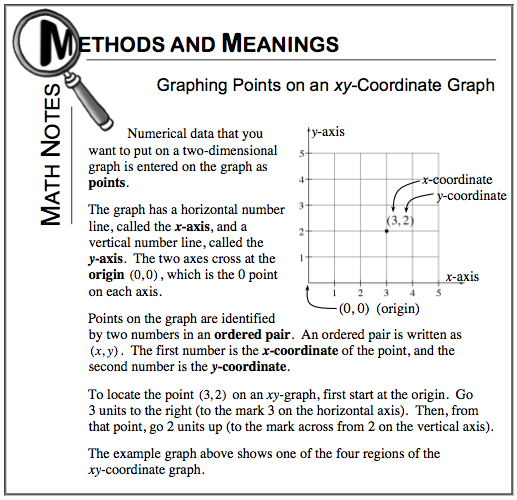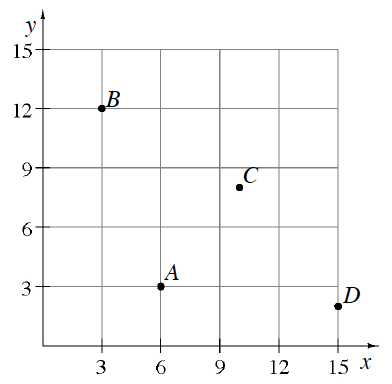### Home > CC1 > Chapter 6 > Lesson 6.1.4 > Problem6-51

6-51.

Name the coordinates of each point shown in the graph at right using ordered pairs $(x, y)$.  Homework Help ✎

Refer to the Math Notes box below to review ordered pairs.A = $(6, 3)$ and B = $(3, 12)$.

Were you able to record the ordered pairs for C and D?